Full Text:<2928>

CLC number: TV131

On-line Access: 2013-04-30

Revision Accepted: 2013-01-21

Crosschecked: 2013-04-19

Cited: 0

Clicked: 6643

Citations:  Bibtex RefMan EndNote GB/T7714

 Journal of Zhejiang University SCIENCE A 2013 Vol.14 No.5 P.362-370 http://doi.org/10.1631/jzus.A1200312

Influence of aeration and initial water thickness on axial velocity attenuation of jet flows*

 Author(s):  Wang-ru Wei1, Jun Deng1, Bin Liu2 Affiliation(s):  1. State Key Laboratory of Hydraulics and Mountain River Engineering, Sichuan University, Chengdu 610065, China; more Corresponding email(s):   wangru_wei@hotmail.com Key Words:  Velocity, Aeration, Initial water thickness, Jet flows Share this article to： More <<< Previous Article|Next Article >>>

Wang-ru Wei, Jun Deng, Bin Liu. Influence of aeration and initial water thickness on axial velocity attenuation of jet flows[J]. Journal of Zhejiang University Science A, 2013, 14(5): 362-370.

@article{title="Influence of aeration and initial water thickness on axial velocity attenuation of jet flows",
author="Wang-ru Wei, Jun Deng, Bin Liu",
journal="Journal of Zhejiang University Science A",
volume="14",
number="5",
pages="362-370",
year="2013",
publisher="Zhejiang University Press & Springer",
doi="10.1631/jzus.A1200312"
}

%0 Journal Article
%T Influence of aeration and initial water thickness on axial velocity attenuation of jet flows
%A Wang-ru Wei
%A Jun Deng
%A Bin Liu
%J Journal of Zhejiang University SCIENCE A
%V 14
%N 5
%P 362-370
%@ 1673-565X
%D 2013
%I Zhejiang University Press & Springer
%DOI 10.1631/jzus.A1200312

TY - JOUR
T1 - Influence of aeration and initial water thickness on axial velocity attenuation of jet flows
A1 - Wang-ru Wei
A1 - Jun Deng
A1 - Bin Liu
J0 - Journal of Zhejiang University Science A
VL - 14
IS - 5
SP - 362
EP - 370
%@ 1673-565X
Y1 - 2013
PB - Zhejiang University Press & Springer
ER -
DOI - 10.1631/jzus.A1200312

Abstract:
With the development of ski-jump energy dissipation for high and large discharge among the hydraulic projects, the effects of characteristics of water flow on energy dissipation are increasingly important. In the present study, the effects of aeration and the initial water thickness on axial velocity attenuation of jet flow were analyzed, using variance analysis and numerical calculated methods. From the analysis of test data, both of the air concentration and initial water thickness are sensitive factors for the axial velocity attenuation of jet flow along the axial way, and there is no significant interaction effect between the aeration and initial water thickness. aeration has a more significant effect on the axial velocity attenuation of jet flow. Decreasing the initial water thickness of jet flow can reduce the length of jet core, and make the initial position of axial velocity attenuation closer to the nozzle exit. The numerical calculation results show that aeration can contribute to the enhancement of entrainment ability of jet flow, which may improve the interaction between jet flow and surroundings. For ski-jump energy dissipation among the hydraulic projects, combining aeration with decreasing initial water thickness of jet flow is an effective way to enhance the rate of axial velocity attenuation.

## 1.  Introduction

Ski-jump energy dissipation prevailed among the hydraulic projects with high head and large discharge located in the alps and valleys, in consideration of funds and project disposal. The characteristics of this type of energy dissipation are that the free jets diffuse and aerate in the air first, and then drop into the pool to form submerged jets, making the energy dissipation happen through disturbance with the surrounding water and air (Fig. 1). This makes the jet flows become water-air two-phase flow with high air concentration. The investigation of aerated jet flow presents many difficulties for experimental research due to the complex two-phase environment.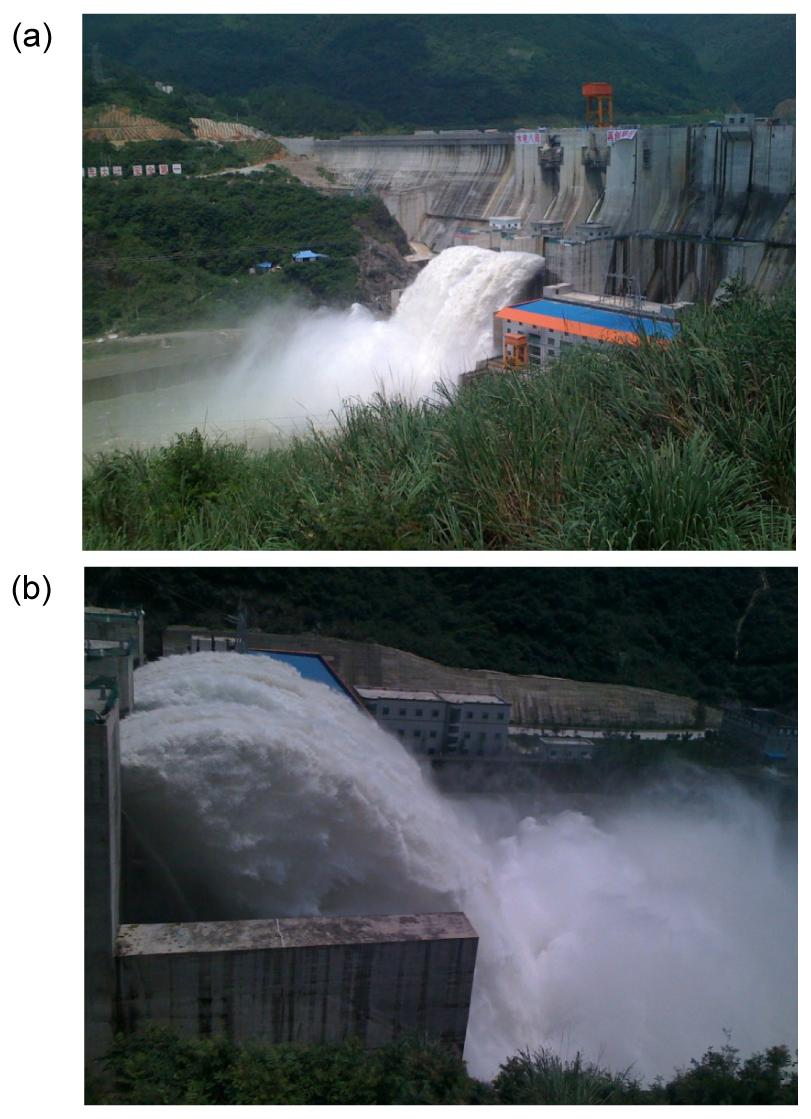Fig.1
Flood discharge with ski-jump flow of Wudu dam spillway in China
(a) Elevation view; (b) Side view

Interest in jet flows is evidenced by the large amount of associated studies (Johnson, ; Afify and Urroz, ; Schmocker et al., ). Chanson et al. () studied air-water flow characteristics close to jet entry with low turbulent intensities. Ervine and Falvey () presented experimental evidence of air concentration decay with different break-up degrees at jet entry. Bohrer et al. () documented velocity decay of free falling rectangular jet in a plunge pool. Due to the difficulties in performing measurements inside the flow, research in this area has concentrated on the resulting outcome of the aerated jet flows, i.e., the impact pressure and the scour in the pool bottom (Chatterjee et al., ; Sarkar and Dey, ; Pagliara et al., ). Regarding jet aeration, the effects of the amount of air on the scouring are much more complicated and the interaction between air-water and riverbed material must be considered, as well as the air entrainment, the geological and geotechnical characteristics of the riverbed. Bollaert and Schleiss (; ; ) investigated plunging jets for a rocky-river bed in facilities, producing jet velocities as high as those encountered in engineering applications. They compared wall pressure measurements at the pool bottom and their propagation inside rock fissures. They also showed that for a rocky-river bed, the air can penetrate rock fissures and trigger stronger eigen-frequency of the fissure. Canepa and Hager () studied the effect of air discharge on plunge pool scour for granular bed materials. They pointed out that for granular bed materials the addition of air to the jet results in an increase of scour depth for a certain water velocity and selected grain characteristics; however, if the reference would have been the air-water mixture velocity, scour depth would have decreased significantly by the addition of air to the jet. In terms of pressure, some research results showed that air entrainment reduces mean impact pressures, but inversely, pressure fluctuations are enhanced with the increasing of air entrainment (Melo, ; Deng et al., ). Manso et al. () presented experimental evidence of plunging jets, using velocities and aeration conditions similar to those in prototype water releasing structures of dams at various pool depths. They stated that impact pressures under the jet have negative skewness in shallow pools and positive skewness in deep pools. Deng et al. (; ) carried out a series of experiments about aerated jet flows with different air concentrations, and indicated that air entrainment could reduce the scour for a granular mixtures riverbed. Xu et al. () showed that dispersing the water flow could increase the shearing area and make the energy diffuse rapidly. Overall, several previous studies have approached different relevant topics for aerated jet flow in plunge pools, but a contrastive analysis of effects caused by aeration and initial water thickness is still missing.

The objective of this paper is to analyze the influence of aeration and initial water thickness on axial velocity attenuation of jet flows. In this paper, the sensitivity of axial velocity attenuation of jet flow for aeration and the initial water thickness of jet flow is experimentally studied. The present study is based on previous research results, and further details and data have been reported in (Wei et al., ). Based on numerical calculations, the effects of aeration and initial water thickness on axial velocity of jet flow are analyzed for further understanding of the characteristics of aerated jet flow.

## 2.  Flow pattern of submerged aerated jet

The submerged aerated jet diffusion is divided into two flow regions: the flow development region, where the jet potential core persists, and the established flow region, based on Hartung and Häusler (), as shown in Fig. 2. In this study, jet centerline travel distance and the section of jet perpendicular to the jet travel distance at the outlet were chosen as the L and r coordinate axes, respectively. The section at the end of flow development region was defined as the initial section of axial velocity attenuation, b is the initial water thickness of jet flow, and V 0 is the velocity at nozzle exit. L 0 is the length of development region. The diffusion spreading angle β is the angle between external boundary and jet axis. For a special section, b e is the distance between external boundary of diffusion and axis of jet flow.

## 3.  Experimental setup and numerical simulation model

Experiments were carried out as shown in Fig. 3.The rectangular glass flume was 3.5 m×0.25 m×0.8 m. The vertical distance from the free water surface to the bottom of the flume was (60±0.2) cm. The nozzle exit was submerged under the free water surface at about 10 cm in the plunge pool. An air-compressor and an air flow-meter were used to provide and control stable air. The air concentration C varied from 0% to 20%. The placement of a honeycomb grid (diameter d 0=1 mm) upstream of the bend was the air entrainment region to improve the homogeneity of the flow over the section. This was a simplified arrangement for the prototype jet flow, and the development of air entrainment of a trajectory jet was neglected. Considering the prototype in spillway flow, the aeration first develops along the spillway which may be set with steps, and then air entrainment occurs during the travel of the jet through the air and the impact with the downstream water cushion (Chanson, ; Manso et al., ; Chanson and Gonzalez, ). The air-water jet flow was almost mixed perfectly, and the mixture air-water flow was considered as a pseudo-fluid where water and air velocities were equal for different air concentrations. The jet outlet had a rectangular nozzle exit of 22 cm width. The initial water thickness of jet flow b changed for 2 cm, 3 cm and 4 cm, by controlling the height of nozzle exit. The incident angle of jet flow was 60°. Eight gauging points were placed along the jet axis from point A to H at intervals of 6 cm. The gauging point A was 1 cm away from the nozzle exit along the jet axis. VA recorded at gauging point A was considered as the initial velocity of jet flow.

Air-water velocity is recorded using CQY-Z8a velocity-meter (China), a double-tip conductivity probe (Fig. 4). The probe consists of two identical tips with an internal concentric electrode made of platinum and an external stainless steel electrode of 0.7 mm diameter. The tips are aligned in the flow direction and the distance between the tips is 13 mm. Both tips are excited by an air bubble detector. This electronic system was designed with a response time less than 10 μs. , where dl is the length between the two probes, and dt is the maximal time where the signal appear.Fig.4
Sketch of the double-tip conductivity probe (unit: mm)

Mixture turbulent model was used to simulate movement of aerated flow, which considers the air-water flow as a uniform mixture flow and contains multiphase with different velocities. The mixture turbulent model is a simplified multiphase flow model. Li et al. () and Chen et al. () showed that the mixture renormalization group (RNG) k-ε double-equation turbulence model is a useful tool in simulating complex water flow. It enables an inter-penetration among different phases and a slip velocity between different phases. The continuity equation, momentum equation, k (turbulent kinetic energy) equation and ε (dissipation rate of turbulent kinetic energy) equation are written as follows:

continuity equation: ,

momentum equation: ,

k-equation: ,

and ε-equation: , where ρ m is the density of the mixed phase of water and air, and u m is the mass averaged velocity. u t and u l are the turbulent viscosity of the mixture phase and the molecular viscosity, respectively. The constants of governing equations C 1ε and C 2ε are 1.44 and 1.92, respectively. Gk ,m is the production term from k- and ε-equation. , , where ρk is the density of k phase, u k is the mass averaged velocity of k phase, αk is the volume fraction of k phase, F is the body force, g is the acceleration of gravity, u eff,m is the coefficient of the virtual viscosity, and u dr,k is the drift velocity of k phase. , , where u kq is the relatively slip velocity between k phase and the first phase (q phase), and f drag is the resistance coefficient.

The structured grids are used to mesh the whole volume for the numerical simulation. To ensure a high accuracy of computation and to reduce the total number of grids, the distribution should comply with the following principles: (1) In order to capture the features of aerated jet flow, the grids near the nozzle exit and along the way should be refined. (2) Limited by the capacity of computer, the grids should be sparse in the region far from the region of jet flow. The aerated jet flows are simulated when the initial velocity at the nozzle exit is 4 m/s with C=0.00, 0.05, 0.10, 0.15, and 0.20. Figs. 5a and 5b show the flow fields for the numerical simulation under different aeration conditions. It is clear that the numerical calculated results are consistent with the pattern of jet flow.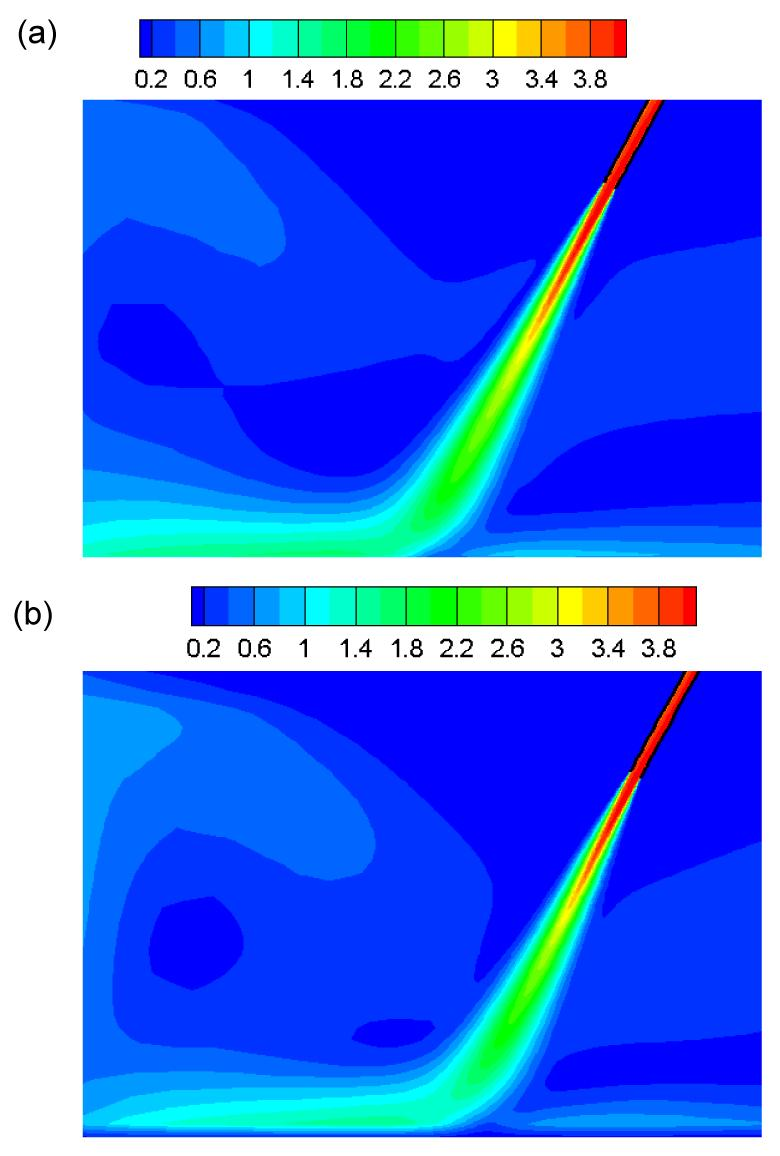Fig.5
Contours of velocity fields of jets from numerical results for different aeration conditions at the initial water thickness of b=2 cm (unit: m/s)
(a) C=0.00; (b) C=0.20

The comparison of the simulated and experimental velocity attenuation along the way is shown in Fig. 6. In this study, the relative jet distance is defined as the ratio of the jet distance L to b, the relative velocity attenuation value is defined as the average velocity VL at each gauging point to the initial velocity V 0 at nozzle exit, where V 0 for the experiments is the value of axial velocity that was measured at the gauging point A. Note that the axial velocity does not always emerge at the same place in the turbulent jet flow and it is hard to obtain the right place of impermanent maximal data measured in the test due to the local experimental condition. This makes the measured data smaller than the real axial velocity of jet flow. It can be seen that, as a whole, the numerical results are basically agreement with the results obtained by experiments.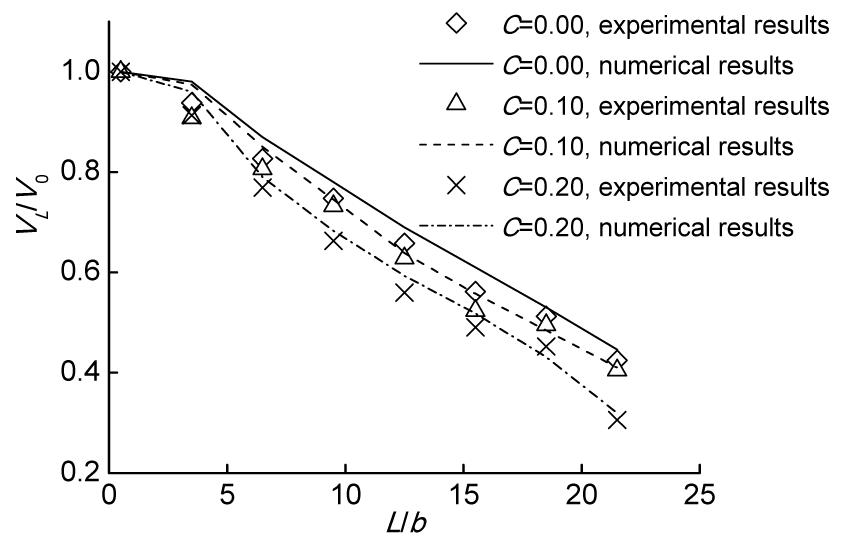Fig.6
Comparison on the velocity attenuation along the way of experimental and numerical results at the initial water thickness of b=2 cm

## 4.  Sensitivity analysis

Adding air to the water flow in the supply system increases the initial velocity (or the kinetic jet energy) of the air-water mixture, compared with the clear water, by continuity property of fluid as the total mixture discharge increases (Manso et al., ). The previous study has shown that when keeping the initial water thickness b and air concentration of jet flow C constant (Wei et al., ), the axial velocity decays for almost the same tendency with the variation of water discharge, indicating that the water discharge or the initial jet velocity has no effects on the axial velocity attenuation of jet flows. Then, when analyzing and comparing different jet aeration, the influence of initial velocity or water discharge is neglected. Previous work results have shown that the axial velocity decreases linearly with the distance along the axial way (Wei et al., ). This attenuation trend becomes faster with the increased air concentration and the decreased initial thickness of jet flow (Fig. 7). The value of slope K for the axial velocity attenuation is defined as the tangent value of the angle between velocity attenuation trend-line and abscissa axis, and the absolute value of slope K is considered as the index for analysis. The absolute values of slope K obtained from experimental data for different conditions are presented in Table 1. It is clear that the absolute value of K increases with the increase of air concentration of jet flow and decrease of the thickness of jet flow at the initial nozzle exit.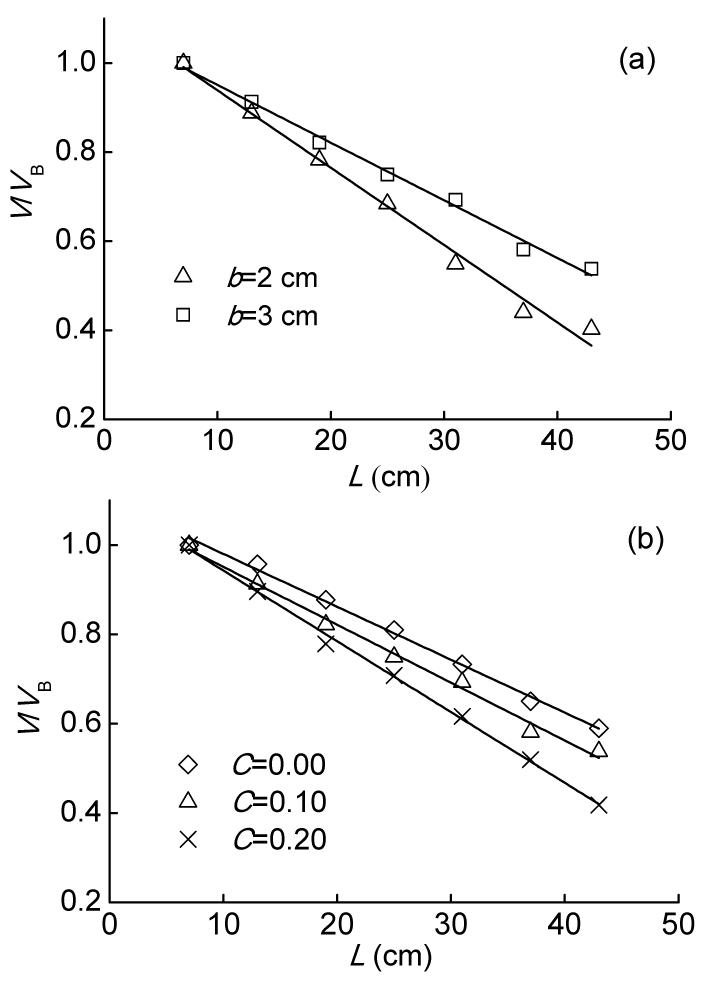Fig.7
Axial velocity attenuation along the axial way from experimental results: the axial velocity attenuation is non-dimensionalized as V/V B
(a) The initial water thickness is 2 cm and 3 cm when air concentration is 0.10; (b) The air concentration is 0.00, 0.10, and 0.20 respectively when the initial water thickness is 3 cm

#### Table 1

Experimental data for different conditions
 Test number C b (cm) K 1 0.00 2 0.0150 2 0.00 3 0.0112 3 0.00 4 0.0091 4 0.05 2 0.0154 5 0.05 3 0.0128 6 0.05 4 0.0111 7 0.10 2 0.0175 8 0.10 3 0.0135 9 0.10 4 0.0126 10 0.20 2 0.0209 11 0.20 3 0.0163 12 0.20 4 0.0143

In physical experimental studies, interaction amongst influencing factors occurs. The experimental results are not only influenced by influencing factors themselves, but also by the combination relationship between different factors. The relationship between K and air concentration of jet flow is shown in Fig. 8a. For a certain initial thickness of jet flow, the K value increases when the air concentration ranged from 0.00 to 0.20. For different initial water thickness of jet flow, the curves did not intersect each other. This indicates that the tendency of axial velocity attenuation caused by aeration does not change noticeably with the different initial water thickness of jet flow. This analogous phenomenon can be seen in Fig. 8b. The tendency of K caused by the initial water thickness has little to do with air concentration of jet flow. The results suggest that there is no interaction between aeration and the initial water thickness of jet flow for the axial velocity attenuation. Therefore, aeration and initial water thickness of jet flow are mutually independent variables, and interaction between aeration and initial water thickness of jet flow does not affect the axial velocity attenuation of jet flow. For the experimental analysis and the hydraulic project design, there is no need to take the interaction between aeration and initial water thickness of jet into consideration.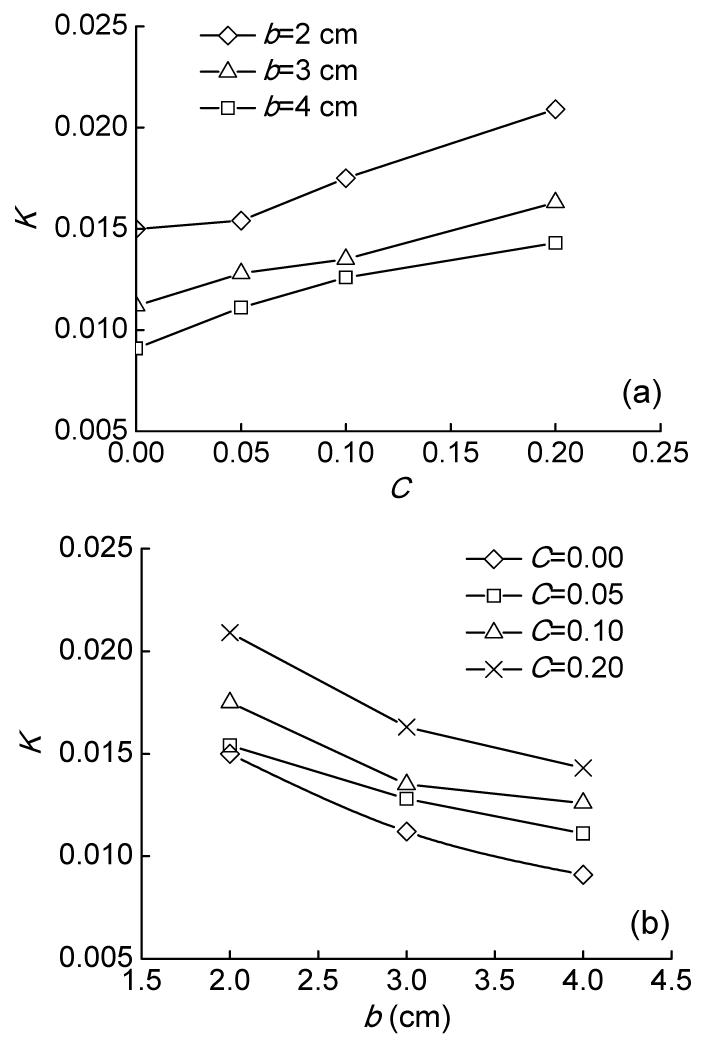Fig.8
Sensitivity analyses on air concentration (a) and initial water thickness (b) from experimental results

The variance analysis of the absolute value of slope K of velocity attenuation is shown in Table 2. The F-test values of b and C are 15.52 and 32.23, respectively. Both F-test values are greater than each F crit, with the significance level α=0.01. This suggests that both aeration and initial water thickness of jet flow have effects on the axial velocity attenuation. Furthermore, for air concentration ranging from 0.0 to 0.2 and the initial water thickness of jet flow changing from 4 cm to 2 cm, the F-test value of C is greater than that of b. This indicates that aeration has more significant effect on the axial velocity attenuation of jet flow than the initial water thickness of jet flow. For ski-jump energy dissipation among the hydraulic projects, improving air entrainment of water flow can be a priority selection.

#### Table 2

Variance analysis of the value of slope K *
 Factor Square of deviance Freedom F F crit (α′=0.01) P b 4.630×10−5 3 15.52 9.78 C 6.412×10−5 2 32.23 10.93 √

• * α′=0.01 is the significance level for the F-test

• ## 5.  Analysis of effects of initial water thickness of jet flow

In jet flow, the axial velocity of jet flow does not decay in the development region of jet flow. The core of a rough turbulent jet flow is not precisely defined, because no direct or exact measurements exist. The core length is often assumed to be about kb. Ervine et al. () indicated an estimate for the core length of about 3–4 diameters for circular jet. Because the core length is direct proportional to initial water thickness of jet flow, the initial attenuation position of axial velocity of jet flow gets closer to the nozzle exit with the decrease of initial water thickness. This can accelerate the velocity attenuation along the axial way. For a certain discharge of jet flow, the water flow can be adjusted to diffuse significantly in either transverse or longitudinal direction when the flow gets out of channel exit. This can decrease the length of development region, as shown in Fig. 9. Therefore, for ski-jump energy dissipation among the hydraulic projects, making the water jets stretched and getting the initial water flow thinner at transverse or longitudinal direction through flip bucket shape optimization is a way to decrease the length of development region and raise the attenuation of axial velocity of jet flow.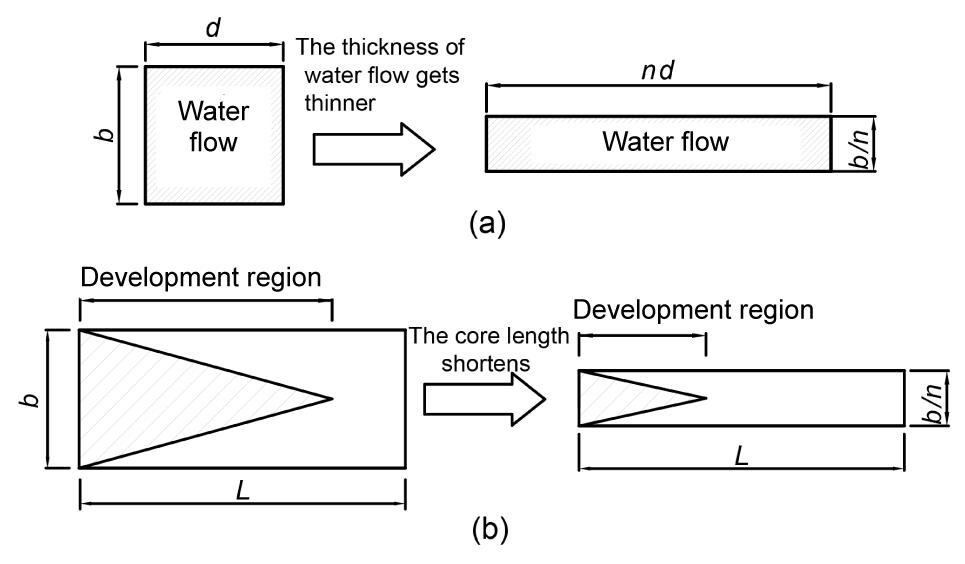Fig.9
Typical pattern of development region in jet flows
(a) Transverse section of water flow; (b) Longitudinal section of water flow

## 6.  Analysis of effects of aeration of jet flow

Albertson et al. () suggested that jet flow diffuses linearly along the way, that is, for a special section, b e increases linearly along the way, and they suggested that the relationship between b e and L can be defined as , with the coefficient c=0.154 when the air concentration of water flow is not aerated.

Assuming that the water flow is incompressible, based on the continuity principle of fluids flow, the increased discharges per unit length should be equivalent to entraining amount of jet flow orthogonal to the axial direction. The entrainment coefficient α is defined as , when c=0.154 suggested by Albertson et al. (), and α=0.067.

For a special section of numerical simulation of jet flow, the external boundary is the position where V r=0.05V mL , where the absolute value of r is b e and V mL is the axial velocity of numerical simulation. It is clear from the numerical results that b e increased linearly along the way, as shown in Fig. 10. When C=0.00, the coefficient c=0.159, which is agreement with the results of Albertson et al. (). When C increases from 0.00 to 0.20, b e becomes larger with the increase of air concentration at a special section. The relationship between C and α at b=2 cm is shown in Fig. 11. With the increase of C from 0.00 to 0.20, α increases from 0.071 to 0.086, about 21.12% larger. This indicates that aeration can improve the enhancement ability of entrainment of jet flow.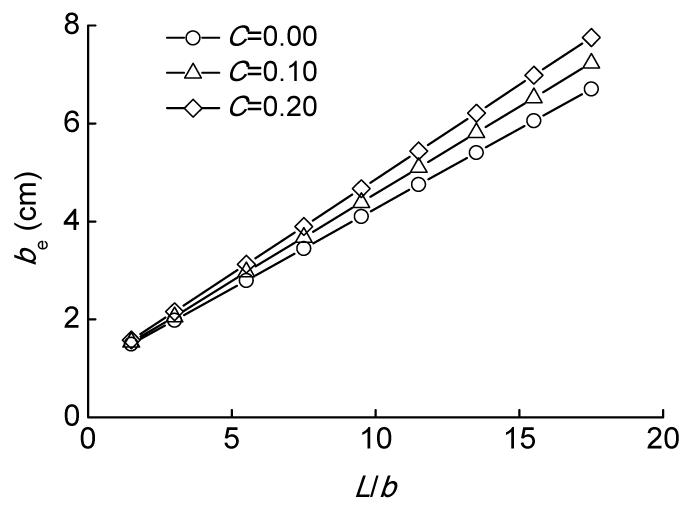Fig.10
Comparison of the value of b e from numerical results for different aeration conditions at the initial water thickness of b=2 cm when the air concentration of jet flow is 0.00 0.10 and 0.20, respectively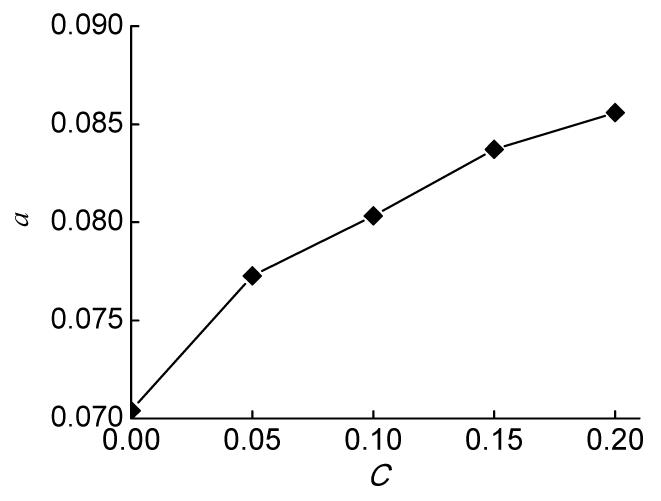Fig.11
Relationship between C and entrainment coefficient α from numerical results at the initial water thickness of b=2 cm

In the present experiments, the interface between the jet flow and surrounding water in the flume was measured, which is considered as the external boundary of jet flow diffusion. Both experimental results and numerical calculated results at the initial water thickness b=2 cm are shown in Fig. 12. It can be seen that the diffusion spreading angle β increases with the increased air concentration of jet flow. This indicates that aeration can improve diffusion of jet flow and the interaction between jet flow and surroundings, and the analysis results of entrainment ability obtained by numerical simulation above is agreement with analysis results shown by the experimental data.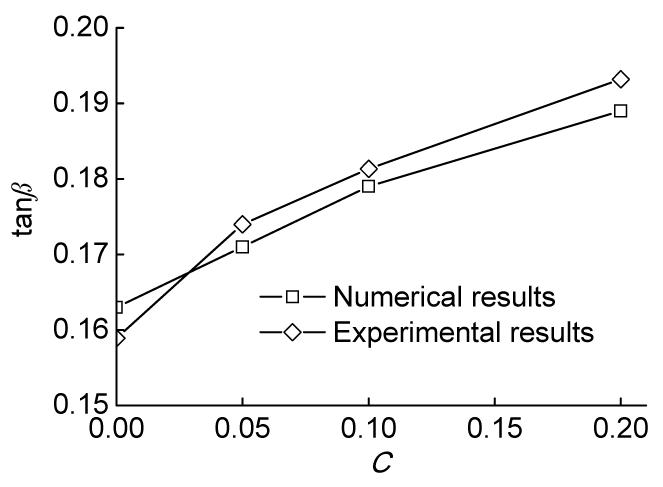Fig.12
Relationship between C and tanβ from experimental and numerical results at the initial water thickness of b=2 cm

## 7.  Discussion

The present study shows that increasing air concentration of jet flows and decreasing the initial water thickness can be considered as efficient ways to improve the axial velocity attenuation of jet flows in downstream river. In the present experimental study, the nozzle exit is submerged under the free water surface at about 10 cm in the plunge pool. This was to make sure that most of the air is entrained into the plunge pool by the initial artificial aeration of jet flow and reduce the influence by plunging of the jet on the air entrainment. Moreover, as shown in Table 1, when the parameters of jet flow change from C=0.00, b=4 cm to C=0.20, b=2 cm, the absolute value of K increases from 0.0091 to 0.0209, about 129.67% larger. It is a remarkable increase for the rate of axial velocity attenuation of jet flow. Therefore, combining aeration with decreasing initial thickness of jet flow is an effective way to enhance the rate of axial velocity attenuation. For ski-jump energy dissipation among the hydraulic projects, the aerators can be set in the spillway artificially to enhance the initial aeration of water flow before the water jets diffuse in the air, and simultaneously, using flaring gate pier, slit-bucket or other types of flip bucket at the spillway outlet to make the free jets stretched at transverse or longitudinal direction in the air, decreasing the length of development region of jet flow and strengthening process of the air entrained into the plunge pool more sufficiently by plunging of the jet. This combination can contribute significantly to the acceleration of axial velocity attenuation of water flow in downstream.

## 8.  Conclusions

A series of experiments was carried out to study the sensitivity of axial velocity attenuation of the jet flow for aeration and initial water thickness. In this paper, when the air concentration changed from 0.00 to 0.20 and the initial thickness of jet flow ranged from 2 cm to 4 cm, there was no significant interaction effect of both of them. The variance analysis results also showed that aeration had more significant effect on the axial velocity attenuation of jet flow than the initial water thickness of jet flow.

The direct proportional relationship between the initial thickness of jet flow and the core length showed that the initial attenuation of axial velocity got closer to the nozzle exit with the decreased initial thickness of jet flow. This could contribute to the acceleration of velocity attenuation along the axial way. Aeration can enhance the enhancement ability of entrainment ability of jet flow, improving the diffusion of jet flow, and may strengthen the interaction between jet flow and surroundings.

For ski-jump energy dissipation in hydraulic projects, the present study showed that increasing air concentration of jet flows and decreasing the initial water thickness can be considered as efficient ways to improve the axial velocity attenuation of jet flows in downstream river. Moreover, the combination of the two, improving the aeration of jet flow and decreasing the initial water thickness simultaneously, can contribute much more significantly to the acceleration of axial velocity attenuation of water flow in downstream.

* Project supported by the National Natural Science Foundation of China (Nos. 51179113, 51009102 and 50909067), and the Program for the New Century Excellent Talents in University (No. NCET-10-0589), China

## ReferencesAfify, A.M., Urroz, G.E., 1994. Plunge-Pool Scour by Inclined Jets. , National Conference on Hydraulic Engineering, New York, USA, 1040-1044. :1040-1044.Albertson, M.L., Dai, Y.B., Jensen, R.A., Rouse, H., 1950. Diffusion of submerged jets. Transactions of the ASCE, 115:639-664.Bohrer, J.G., Abt, S.R., Wittler, R.J., 1998. Predicting plunge pool velocity decay of free falling rectangular jet. Journal of Hydraulic Engineering, ASCE, 124(10):1043-1048.Bollaert, E., Schleiss, A., 2003. Scour of rock due to the impact of plunging high velocity jets. Part I: A state-of-the-art review. Journal of Hydraulic Research, 41(5):451-464.Bollaert, E., Schleiss, A., 2003. Scour of rock due to the impact of plunging high velocity jets. Part II: Experimental results of dynamic pressures at pool bottoms and in one- and two-dimensional closed end rock joints. Journal of Hydraulic Research, 41(5):465-480.Bollaert, E., Schleiss, A., 2005. Physically based model for evaluation of rock scour due to high-velocity jet impact. Journal of Hydraulic Engineering, ASCE, 131(3):153-165.Canepa, S., Hager, W.H., 2003. Effect of jet air content on plunge pool scour. Journal of Hydraulic Engineering, ASCE, 129(5):358-365.Chanson, H., 1997. Air bubble entrainment in open channels: flow structure and bubble size distributions. International Journal of Multiphase Flow, 23(1):193-203.Chanson, H., Gonzalez, C.A., 2005. Physical modeling and scale effects of air-water flows on stepped spillway. Journal of Zhejiang University SCIENCE, 6A(3):243-250.Chanson, H., Aoki, S., Hoque, A., 2004. Physical modeling and similitude of air bubble entrainment at vertical circular plunging jets. Chemical Engineering Science, 59(4):747-758.Chatterjee, S.S., Ghosh, S.N., Chatterjee, M., 1994. Local scour due to submerged horizontal jet. Journal of Hydraulic Engineering, ASCE, 120(8):973-992.Chen, J.G., Zhang, J.M., Xu, W.L., Wang, Y.R., 2010. Numerical simulation of the energy dissipation characteristics in stilling basin of multi-horizontal submerged jets. Journal of Hydrodynamics, 22(5):71-80.Deng, J., Xu, W.L., Qu, J.X., 2002. Influence of aeration on scouring. Journal of Hydraulic Engineering, (in Chinese),10:8-13.Deng, J., Xu, W.L., Zhang, J.M., Wang, W., Liu, S.J., 2008. A new type of plunge pool—Multi-horizontal submerged jets. Science in China Series E-Technological Sciences, 51(12):2128-2141.Deng, J., Xu, W.L., Liu, S.J., Wang, W., 2009. Influence of water jet aeration on pressure in scour pool and plunge pool. Advances in Water Science, (in Chinese),20(3):373-378.Ervine, D.A., Falvey, H.T., 1987. Behaviour of turbulent water jets in the atmosphere and in punge pools. ICE Proceedings, 83(1):295-314.Ervine, D.A., Falvey, H.T., Withers, W., 1997. Pressure fluctuations on plunge pool floors. Journal of Hydraulic Research, 35(2):257-279.Hartung, F., Husler, E., 1973. Scours, still Basins and Downstream Protection under Free Overfall Jets at Dams. , The 11th Congress on Large Dams, Madrid, Spain, 39-56. :39-56.Johnson, G., 1967. The Effects of Entrained Air on the Scouring Capacity of Water Jets. , The 12th Congress of IAHR, Fort Collins, USA, 218-226. :218-226.Li, Z.W., Huai, W.X., Qian, Z.D., 2009. Numerical simulation of turbulent radial jets in static ambient. Journal of Hydraulic Engineering, (in Chinese),40(11):1320-1325.Manso, P.A., Fiorotto, V., Bollaert, E., Schleiss, A.J., 2004. Discussion of “Effect of Jet Content on Plunge Pool Scour” by Stefano Canepa and Willi H. Hager. Journal of Hydraulic Engineering, ASCE, 130(11):1128-1130.Manso, P.A., Bollaert, E.F.R., Schleiss, A.J., 2007. Impact pressures of turbulent high-velocity jets plunging in pools with flat bottom. Experiments in Fluids, 42(1):49-60.Melo, J.F., 2002. Reduction of Plunge Pool Floor Dynamic Pressure due to Jet Air Entrainment. Rock Scour due to Falling High-Velocity Jets. , Proceedings of the International Workshop, Lausanne, Switzerland, 125-136. :125-136.Pagliara, S., Hager, W.H., Minor, H.E., 2005. Hydraulics of plane plunge pool scour. Journal of Hydraulic Engineering, ASCE, 132(5):450-461.Sarkar, A., Dey, S., 2004. Review on local scour due to jets. International Journal of Sediment Research, 19(3):210-238.Schmocker, L., Pfister, M., Hager, W.H., 2008. Aeration characteristics of ski jump jets. Journal of Hydraulic Engineering, ASCE, 134(1):90-97.Wei, W.R., Deng, J., Liu, B., 2011. Experimental investigation on the effects of aeration on the velocity attenuation of jet flow. Journal of Sichuan University, (in Chinese),43(supp.1):29-33.Xu, W.L., Liao, H.S., Yang, Y.Q., 1998. Computational and experimental investigation on the 3-d flow feature and energy dissipation characteristics of plunge pools. Journal of Theoretical and Applied Mechanic, 30(1):35-42.